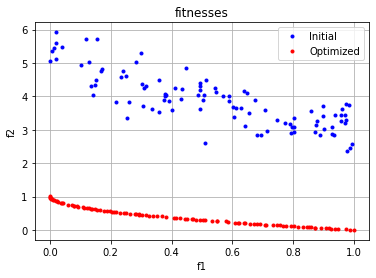# [PYTHON] I implemented NSGA-II, a multi-objective optimization problem.

• Articles sent by data scientists from the manufacturing industry
• This time, NSGA-II was implemented (using sample code) in the multi-purpose optimization method.

## Introduction

I will introduce the multi-purpose optimization (NSGA-II) that I introduced the other day. What is Multi-Objective Optimization, NSGA-II? Please refer to the above article for more information.

## Library to use (deap)

This time, I would like to implement it using the genetic algorithm library deap. There are other libraries, but this time I will use deap, which seems to be under active development.

## Implementation of NSGA-II

This time, there was a sample of NSGA-II in the tutorial of deap, so I used that.

The python code is below.

``````#Import required libraries
import array
import random
import json

import numpy as np
import matplotlib.pyplot as plt

from math import sqrt

from deap import algorithms
from deap import base
from deap import benchmarks
from deap.benchmarks.tools import diversity, convergence, hypervolume
from deap import creator
from deap import tools
``````

First, we will design the genetic algorithm.

``````#Creating a goodness-of-fit class that is optimized by minimizing the goodness of fit
creator.create("FitnessMin", base.Fitness, weights=(-1.0, -1.0))
#Create individual class Individual
creator.create("Individual", array.array, typecode='d', fitness=creator.FitnessMin)
#Creating a Toolbox
toolbox = base.Toolbox()
#Specify the range of values ​​that a gene can take
BOUND_LOW, BOUND_UP = 0.0, 1.0
#Specify the number of genes in one individual
NDIM = 30

#Gene generation function
def uniform(low, up, size=None):
try:
return [random.uniform(a, b) for a, b in zip(low, up)]
except TypeError:
return [random.uniform(a, b) for a, b in zip([low] * size, [up] * size)]

#Functions that generate genes"attr_gene"Register
toolbox.register("attr_float", uniform, BOUND_LOW, BOUND_UP, NDIM)
#Function to generate an individual "individual""Register
toolbox.register("individual", tools.initIterate, creator.Individual, toolbox.attr_float)
#Functions that generate populations"population"Register
toolbox.register("population", tools.initRepeat, list, toolbox.individual)
#Evaluation function"evaluate"Register
toolbox.register("evaluate", benchmarks.zdt1)
#Function to cross"mate"Register
toolbox.register("mate", tools.cxSimulatedBinaryBounded, low=BOUND_LOW, up=BOUND_UP, eta=20.0)
#Mutant function"mutate"Register
toolbox.register("mutate", tools.mutPolynomialBounded, low=BOUND_LOW, up=BOUND_UP, eta=20.0, indpb=1.0/NDIM)
#Individual selection method"select"Register
toolbox.register("select", tools.selNSGA2)
``````

In deap, an individual can be treated as an individual by creating a data type that extends the list type. Since what is being done in each code is described so that it can be understood in the comment text, please forgive the part where the entire code is difficult to see.

This time, there are two objective functions, and both are set to minimize the problem. deap uses a minimum of "-1" and a maximum of "1".

Next is the actual evolutionary computation part.

``````def main():
random.seed(1)

NGEN = 250 #Number of repeating generations
MU = 100 #Population in the population
CXPB = 0.9 #Crossover rate

#Setting what to output to the log during the generation loop
stats = tools.Statistics(lambda ind: ind.fitness.values)
stats.register("min", np.min, axis=0)
stats.register("max", np.max, axis=0)

logbook = tools.Logbook()
logbook.header = "gen", "evals", "std", "min", "avg", "max"

#First generation generation
pop = toolbox.population(n=MU)
pop_init = pop[:]
invalid_ind = [ind for ind in pop if not ind.fitness.valid]
fitnesses = toolbox.map(toolbox.evaluate, invalid_ind)
for ind, fit in zip(invalid_ind, fitnesses):
ind.fitness.values = fit
pop = toolbox.select(pop, len(pop))

record = stats.compile(pop)
logbook.record(gen=0, evals=len(invalid_ind), **record)
print(logbook.stream)

#Performing optimal calculations
for gen in range(1, NGEN):
#Population generation
offspring = tools.selTournamentDCD(pop, len(pop))
offspring = [toolbox.clone(ind) for ind in offspring]

#Crossover and mutation
for ind1, ind2 in zip(offspring[::2], offspring[1::2]):
#Select individuals to cross
if random.random() <= CXPB:
#Crossing
toolbox.mate(ind1, ind2)

#mutation
toolbox.mutate(ind1)
toolbox.mutate(ind2)

#Crossed and mutated individuals remove fitness
del ind1.fitness.values, ind2.fitness.values

#Re-evaluate fitness for individuals with fitness removed
invalid_ind = [ind for ind in offspring if not ind.fitness.valid]
fitnesses = toolbox.map(toolbox.evaluate, invalid_ind)
for ind, fit in zip(invalid_ind, fitnesses):
ind.fitness.values = fit

#Select the next generation
pop = toolbox.select(pop + offspring, MU)
record = stats.compile(pop)
logbook.record(gen=gen, evals=len(invalid_ind), **record)
print(logbook.stream)

#Output the last generation hyper volume
print("Final population hypervolume is %f" % hypervolume(pop, [11.0, 11.0]))

return pop, pop_init, logbook
``````

With a general genetic algorithm, you may feel that the crossover rate of 0.9 (CXPB = 0.9) is high. However, since NSGA-II preserves the parent population, it is recognized that the crossover rate is high and there is no problem. Personally, I think that the crossover rate may be 1.0.

After that, call this main function.

``````if __name__ == '__main__':

pop, pop_init, stats = main()
``````

This time, I would like to visualize the results of the initial sample and the final generation.

``````fitnesses_init = np.array([list(pop_init[i].fitness.values) for i in range(len(pop_init))])
fitnesses = np.array([list(pop[i].fitness.values) for i in range(len(pop))])
plt.plot(fitnesses_init[:,0], fitnesses_init[:,1], "b.", label="Initial")
plt.plot(fitnesses[:,0], fitnesses[:,1], "r.", label="Optimized" )
plt.legend(loc="upper right")
plt.title("fitnesses")
plt.xlabel("f1")
plt.ylabel("f2")
plt.grid(True)
``````## at the end

Thank you for reading to the end. This time, I checked the sample code for NSGA-II, which is a multi-purpose optimization.

If you have a request for correction, we would appreciate it if you could contact us.